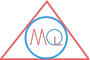# Area and Perimeter of Triangle

## Perimeter of triangle

Perimeter is the length of boundary around any closed figure.

The boundary of a triangle consists of its three sides. Therefore, sum of length of three sides of triangle is called its perimeter.

### What are the units of perimeter?

Units of perimeter measurement of a triangle is taken same as units of length of sides of the triangle.

The few examples of units of length of side of triangle are meter (m), centimeter (cm) etc.. So, the units of perimeter are also taken as meter (m), centimeter (cm) etc..

### How to calculate perimeter of a triangle?

Perimeter of triangle $$\triangle ABC$$In this $$\triangle ABC$$, we have

length of AB = a

length of BC = b

and length of CA = c

So, perimeter of $$\triangle ABC$$ is sum of length of sides AB, BC and CA

i.e. perimeter of $$\triangle ABC$$ = $$a + b + c$$

## Perimeter of an equilateral triangle

Equilateral triangle $$\triangle ABC$$ with sides length aIn equilateral triangle, where all sides are of equal length.

i.e. AB = a, BC = a and CA = a

So, perimeter of equilateral $$\triangle ABC$$ = $$a + a + a = 3a$$

## Perimeter of isosceles triangle

Isosceles triangle $$\triangle ABC$$ with sides length a, b and aIn isosceles triangle, where any two sides are of equal length.

i.e. AB = a, BC = a and CA = b

So, perimeter of isosceles $$\triangle ABC$$ = $$a + a + b = 2a + b$$

Example

Find perimeter of $$\triangle ABC$$ with length of its sides as given below:

AB=2cm, BC=4cm, CA=6cm

So, perimeter of $$\triangle ABC$$ = $$AB + BC + CA$$

= $$2 + 4 + 6$$

= $$12$$cm

## Area of triangle

Area is the total amount of space occupied by any closed figure.

### What are the units of area?

Area is measured in square units.

The few examples of units of area of triangle are $$meter^2$$ or ($$m^2$$) read as meter square, $$centimeter^2$$ ($$cm^2$$) read as centimeter square etc..

## How to calculate area of a triangle?

Area of any triangle = $$\frac{1}{2} \times base \times height$$

Let’s understand it from following $$\triangle ABC$$

Area of triangle $$\triangle ABC$$where, BC is base, length of BC = b

and AO is height, length of AO = h

Therefore, area of $$\triangle ABC$$ = $$\frac{1}{2} \times b \times h$$

## Area of equilateral triangle

Area of equilateral triangle $$\triangle ABC$$In above equilateral $$\triangle ABC$$, where all sides of triangle are equal in length, its area is calculated as:

area of $$\triangle ABC$$ = $$\frac{\sqrt 3}{4} \times a^2$$

Let’s see how it is calculated?

Here, in right angle $$\triangle AOC$$

$$(a)^2 = (h)^2 + (\frac{a}{2})^2$$          by Pythagoras theorem

$$a^2 = h^2 + \frac{a^2}{4}$$

$$a^2 – \frac{a^2}{4} = h^2$$

$$\frac{4a^2 – a^2}{4} = h^2$$

$$\frac{3a^2}{4} = h^2$$

$$\frac{\sqrt 3}{2}a = h$$

Area of $$\triangle = \frac{1}{2} \times a \times \frac{\sqrt 3}{2}a$$

Area of $$\triangle = \frac{\sqrt 3}{4}a^2$$

## Area of isosceles triangle

Area of isosceles triangle $$\triangle ABC$$area of isosceles $$\triangle ABC = \frac{b\sqrt{4a^2-b^2}}{4}$$

Let’s see how it is calculated?

Here, in right angle $$\triangle AOC$$

$$(a)^2 = (h)^2 + (\frac{b}{2})^2$$          by Pythagoras theorem

$$a^2 = h^2 + \frac{b^2}{4}$$

$$a^2 – \frac{b^2}{4} = h^2$$

$$\frac{4a^2 – b^2}{4} = h^2$$

$$\sqrt \frac{4a^2 – b^2}{4} = h$$

$$\frac{\sqrt{4a^2-b^2}}{2} = h$$

we know, area of $$\triangle = \frac{1}{2} \times b \times h$$

$$\therefore$$ area of $$\triangle ABC = \frac{1}{2} \times b \frac{\sqrt{4a^2-b^2}}{2}$$

or area of $$\triangle ABC = \frac{b\sqrt{4a^2-b^2}}{4}$$